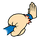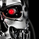1945 lượt xem
1945
Quarters Theory v2
Quarters Theory automatically plotted for you on your chart. This theory is primary on forex pairs. Major quarters, Minor quarters, quarters, and hesitation zones all plotting. Please refer to the inputs on their color. (still having a fill issues with the hesitation zone)
```//@version=2
study("Quarters", overlay = true)
//based on Quarters Theory this is based mostly for forex trading applications
//Major Quarters every 250 pips
//Minor Quarters every 125 pips
//Quarters every 25 pips
//Hesitation zones based on a +-75 pips of a major quarter

FirstBar = barstate.isrealtime//calculating everything backwards so we don't have repainting issues plots on the current bar
//top1 = input(defval=105, type = float,title = "level of Major/Minor Quarter confluence value on the chart", confirm = true)
sq = input(true, title = "Show Quarters")
sm =  input(true, title = "Show Minor Quarters")
ShowM =  input(true, title = "Show Major Quarters")
hz = input(true, title = "Show Hesitation Zones")
topprice = iff(FirstBar and highest(high,250),high,na)//high <= highest(high,50), high, na)// na(topprice) ? high : high >= topprice ? high : topprice //valuewhen(high > highest(high,200),high,1)  //
botprice = iff(FirstBar and lowest(low,250),low,na) //low <= lowest(low,50), low, na)//na(botprice) ? low : low <= botprice ? low : botprice   //valuewhen(low > lowest(low,200),low,1)
range = ((topprice - botprice) * .5)
anchor = botprice + range
primaryanchor = round(anchor / syminfo.pointvalue) //round(anchor / syminfo.pointvalue)
Major_q_increment = (2.5 * syminfo.pointvalue)

// creates Top Major quarters based on the highest value
Major_Plus2 = primaryanchor + (2 * Major_q_increment)
Major_Plus1 = primaryanchor + Major_q_increment
Major_line = primaryanchor
Major_Minus1 = primaryanchor - Major_q_increment
Major_Minus2 = primaryanchor - (2 * Major_q_increment)

Minor_q_increment = (1.25 * syminfo.pointvalue)

//calculates Top Minor quarters based on the highest value
Minor_Plus2 = primaryanchor + (2* Minor_q_increment)
Minor_Plus1 = primaryanchor + Minor_q_increment
Minor_Minus1 = primaryanchor - Minor_q_increment
Minor_Minus2 = primaryanchor - (2 * Minor_q_increment)

//calculates out the current quarter
current_quarter = round((close * syminfo.pointvalue))
Quarters_increment = (.25 * syminfo.pointvalue)

//calculates quarters from top value +5 on each side
Quarters_Plus5 = current_quarter + (4* Quarters_increment)
Quarters_Plus4 = current_quarter + (3* Quarters_increment)
Quarters_Plus3 = current_quarter + (2* Quarters_increment)
Quarters_Plus2 = current_quarter + ( Quarters_increment)
Quarters_1 = current_quarter
Quarters_Minus2 = current_quarter - ( Quarters_increment)
Quarters_Minus3 = current_quarter - (2 * Quarters_increment)
Quarters_Minus4 = current_quarter - (3 * Quarters_increment)
Quarters_Minus5 = current_quarter - (4 * Quarters_increment)

//calculates the hesitation zone
hestitation_increment = .75 * syminfo.pointvalue
Hest_2T = Major_Plus2 + hestitation_increment
Hest_2B = Major_Plus2 - hestitation_increment
Hest_1T = Major_Plus1 + hestitation_increment
Hest_1B = Major_Plus1 - hestitation_increment
Hest_BT = Major_line + hestitation_increment
Hest_BB = Major_line - hestitation_increment
Hest_L1T = Major_Minus1 + hestitation_increment
Hest_L1B = Major_Minus1 - hestitation_increment
Hest_L2T = Major_Minus2 + hestitation_increment
Hest_L2B = Major_Minus2 - hestitation_increment

//plots the major quarters
plot(ShowM and FirstBar ? Major_Plus2: na,"MLP2", color= blue, linewidth = 3, style = circles, trackprice = true)
plot(ShowM and FirstBar ? Major_Plus1: na,"MLP1", color= blue, linewidth = 3, style = circles, trackprice = true)
plot(ShowM and FirstBar ? Major_line: na,"MLD2", color= purple, linewidth = 3, style = circles, trackprice = true)
plot(ShowM and FirstBar ? Major_Minus1: na,"MM1", color= blue, linewidth = 3, style = circles, trackprice = true)
plot(ShowM and FirstBar ? Major_Minus2: na,"MM2", color= blue, linewidth = 3, style = circles, trackprice = true)

//plot the minor quarters
plot(sm and FirstBar ? Minor_Plus2 : na,"MP1", color= orange, linewidth = 2, trackprice = true)
plot(sm and FirstBar ? Minor_Plus1 : na,"MP1", color= orange, linewidth = 2, trackprice = true)
plot(sm and FirstBar ? Minor_Minus1 : na,"Mm1", color= orange, linewidth = 2, trackprice = true)
plot(sm and FirstBar ? Minor_Minus2 : na,"Mm2", color= orange, linewidth = 2, trackprice = true)

//plots the quarters
plot(sq and FirstBar ? Quarters_Plus5: na,"qp5", color= Quarters_Plus5 != Quarters_Plus5 ? gray: na, linewidth = 1, trackprice = true)
plot(sq and FirstBar ? Quarters_Plus4: na,"qp4", color= Quarters_Plus4 != Quarters_Plus4 ? gray: na, linewidth = 1, trackprice = true)
plot(sq and FirstBar ? Quarters_Plus3: na,"qp3", color= Quarters_Plus3 != Quarters_Plus3 ? gray: na, linewidth = 1, trackprice = true)
plot(sq and FirstBar ? Quarters_Plus2: na,"qp2", color= Quarters_Plus2 != Quarters_Plus2 ? gray: na, linewidth = 1, trackprice = true)
plot(sq and FirstBar ? current_quarter: na,"q",color= current_quarter != current_quarter ? gray: na, linewidth = 1, trackprice = true)
plot(sq and FirstBar ? Quarters_Minus2: na,"qm2",color= Quarters_Minus2 != Quarters_Minus2 ? gray: na, linewidth = 1, trackprice = true)
plot(sq and FirstBar ? Quarters_Minus3: na,"qm3", color= Quarters_Minus3 != Quarters_Minus3 ? gray: na, linewidth = 1, trackprice = true)
plot(sq and FirstBar ? Quarters_Minus4: na,"qm4", color= Quarters_Minus4 != Quarters_Minus4 ? gray: na, linewidth = 1, trackprice = true)
plot(sq and FirstBar ? Quarters_Minus5: na,"qm5", color= Quarters_Minus5 != Quarters_Minus5 ? gray: na, linewidth = 1, trackprice = true)

//plots hestitation zone
HH2T = plot(hz and FirstBar ? Hest_2T: na,"H2T", linewidth = 1, color= gray, trackprice = true)
HH2B = plot(hz and FirstBar ? Hest_2B: na,"H2B", linewidth = 1, color= gray, trackprice = true)
HH1T = plot(hz and FirstBar ? Hest_1T: na,"H1T", linewidth = 1, color= gray, trackprice = true)
HH1B = plot(hz and FirstBar ? Hest_1B: na,"H1B", linewidth = 1, color= gray, trackprice = true)
HHT = plot(hz and FirstBar ? Hest_BT: na,"MT", linewidth = 1, color= gray, trackprice = true)
HHB = plot(hz and FirstBar ? Hest_BB: na,"MB", linewidth = 1, color= gray, trackprice = true)
HL1T = plot(hz and FirstBar ? Hest_L1T: na,"L1T", linewidth = 1, color= gray, trackprice = true)
HL1B = plot(hz and FirstBar ? Hest_L1B: na,"L1B", linewidth = 1, color= gray, trackprice = true)
HL2T = plot(hz and FirstBar ? Hest_L2T: na,"L2T", linewidth = 1, color= gray, trackprice = true)
HL2B = plot(hz and FirstBar ? Hest_L2B: na,"L2B", linewidth = 1, color= gray, trackprice = true)

fill(HH2T, HH2B, color = gray, transp = 50, title = "Major 2 Top zone", editable = true)
fill(HH1T, HH1B, color = gray, transp = 50, title = "Major 1 Top zone", editable = true)
fill(HHT, HHB, color = gray, transp = 50, title = "Major Primary zone", editable = true)
fill(HL1T, HL1B, color = gray, transp = 50, title = "Major 1 Low zone", editable = true)
fill(HL2T, HL2B, color = gray, transp = 50, title = "Major 2 Low zone", editable = true)
```

## Bình luậnlenes
@lenes, I take it @Gqman hasn't updated or fixed this issue? Seems it has something to do with the differences in point values that are at 1.xxxx or 0.xxxx.

I will be editing the code to correct the issue and will repost my revised code later.
Phản hồiThis is great. Not a lot of people know about this theory. Surprised to find it here.
Phản hồi